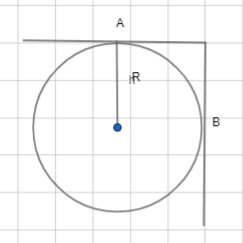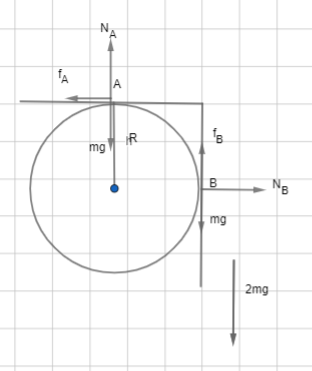QuestionAnswers

# A rod bent at right angle along its centre line, is placed on a rough horizontal fixed cylinder of radius R as shown in the figure. Mass of the rod is 2m and the rod is in equilibrium. Assume that the friction force on rod at A and B is equal in magnitudeThis question has multiple correct optionsA. Normal force applied by cylinder on rod at A is $3mg/2$B. Normal force applied by cylinder on rod at B must be zeroC. Friction force acting on rod at B is upwardD. Normal force applied by cylinder on rod at A is mgHint: Draw a free body diagram according to the question and show all the components of the force. Then equate all the force components and solve the equations to find the forces which are asked in the option and compare the results to see which option is true.

First we will draw the component of various forces at point A and B.At point A we have the normal force, weight and the friction as ${{N}_{A}},mg,{{f}_{A}}$ respectively and point B we have the normal force, weight and the friction as ${{N}_{B}},mg,{{f}_{B}}$ respectively.
Now we have the total weight of the rod is 2mg. so, equating the downward weight with the upward forces we get,
${{N}_{A}}+{{f}_{B}}=2mg\text{ }\to \text{ 1}$
Now comparing the horizontal forces, we get,
${{f}_{A}}={{N}_{B}}\text{ }\to \text{ 2}$
Now balancing the torque of the rod with respect to the centre, we can write,
\begin{align} & {{f}_{A}}R+{{f}_{B}}R=mgR \\ & {{f}_{A}}+{{f}_{B}}=mg\text{ }\to \text{ 3} \\ \end{align}
Again, from the question we have that,
${{f}_{A}}={{f}_{B}}\text{ }\to \text{ 4}$
Now, comparing the equation 3 and equation 4, we get,
${{f}_{A}}={{f}_{B}}=\dfrac{mg}{2}$
Putting this value on equation 2, we get,
${{N}_{B}}=\dfrac{mg}{2}$
Again, from equation 1 we get,
${{N}_{A}}=\dfrac{3mg}{2}$
From these values, we can say that the normal force applied by the cylinder to the rod at point A is $\dfrac{3mg}{2}$. So, option A is true.
Normal force at point B is not zero. Option B is wrong.
Friction force on rod at point B is upward. Option C is correct.
Option D is wrong since normal force at A is $\dfrac{3mg}{2}$.
So, the correct options are (A), (C).
Note: The normal force to a body will not be always in the opposite direction to the weight of the body. The normal force will be in the opposite direction to the force the body puts on the surface it is in and is perpendicular to the surface.

View Notes
Phase AngleQuantum MechanicsCBSE Class 11 Physics Waves FormulasCBSE Class 11 Physics Oscillations FormulasCBSE Class 11 Physics Thermodynamics FormulasCBSE Class 11 Physics Gravitation FormulasElectric Current and Its EffectsCBSE Class 11 Physics Kinetic Theory FormulasCBSE Class 11 Physics Law of Motion FormulasQuantum Mechanics Used in Real LifeImportant Questions for CBSE Class 11 Physics Chapter 3 - Motion in a Straight LineNCERT Books Free Download for Class 11 Physics Chapter 3 - Motion in a Straight LineImportant Questions for CBSE Class 11 Physics Chapter 4 - Motion in a PlaneImportant Questions for CBSE Class 11 PhysicsImportant Questions for CBSE Class 11 Physics Chapter 12 - ThermodynamicsImportant Questions for CBSE Class 11 Physics Chapter 14 - OscillationsImportant Questions for CBSE Class 11 English Snapshots Chapter 4 - Albert Einstein at SchoolImportant Questions for CBSE Class 11 Physics Chapter 11 - Thermal Properties of MatterImportant Questions for CBSE Class 11 Physics Chapter 15 - WavesImportant Questions for CBSE Class 11 Physics Chapter 8 - GravitationCBSE Class 12 Physics Question Paper 2020CBSE Class 10 Hindi A Question Paper 2020Hindi A Class 10 CBSE Question Paper 2009Hindi A Class 10 CBSE Question Paper 2015Hindi A Class 10 CBSE Question Paper 2007Hindi A Class 10 CBSE Question Paper 2013Hindi A Class 10 CBSE Question Paper 2016Hindi A Class 10 CBSE Question Paper 2012Hindi A Class 10 CBSE Question Paper 2010Previous Year Question Paper for CBSE Class 12 PhysicsNCERT Exemplar for Class 11 Physics Chapter 3 - Motion in a Straight Line (Book Solutions)NCERT Solutions for Class 11 Physics Chapter 3 Motion in a Straight Line in HindiClass 11 Physics NCERT solutionsHC Verma Class 11 Physics Part-1 Solutions for Chapter 9 - Centre of Mass, Linear Momentum, CollisionRS Aggarwal Class 6 Solutions Chapter-11 Line Segment, Ray and LineHC Verma Class 11 Physics Part-1 Solutions for Chapter 13 - Fluid MechanicsNCERT Solutions for Class 11 Physics Chapter 4 – Motion In a PlaneNCERT Solutions for Class 11 Physics Chapter 4 Motion in a Plane In HindiRS Aggarwal Solutions Class 6 Chapter-11 Line Segment, Ray and Line (Ex 11A) Exercise 11.1NCERT Exemplar for Class 11 Physics Chapter 4 - Motion in a Plane (Book Solutions)